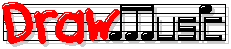Pop Music Theory

Lessons in Order

 1-7 (Intro & Pitch) +
 8-18 (Major Scale) +
 19-29 (Chord Progressions) -
 30-34 (Hook Chords) +
 35-41 (Written Notes) +
 42-50 (Song Chorus) +

Lessons by Topic

 Strategy +
 Pitches +
 Scales +
 Written Notes +
 Chords +
 Chord Progressions +
 Melody +
 Songwriting Steps +
 Science +
 Games & Tools +
 Song Examples: Crazy +
 Song Examples: Rolling Stone +

Detailed Contents

Lesson 21: Scales Above 8

This lesson teaches the major scale degrees above degree 8 (degrees 9, 10, 11, etc.).

Before taking this lesson, you should know:

You'll need these extended scale degrees to understand various theory topics, such as Lesson 22: Diatonic Triads.

You know that the common scales each have 7 different pitches or degrees, plus degree 8, which is just the same pitch as degree 1 an octave higher. We can keep going above degree 8 as far as we like, with scale degrees 9, 10, 11, etc.

These extended scale degrees do the same thing that degree 8 does; they reuse the same pitch-names as lower scale degrees. Here's an extended B♭ major scale as an example:

So, these pairs of scale degrees share the same pitch names:

Degree 8 = degree 1
Degree 9 = degree 2
Degree 10 = degree 3
Degree 11 = degree 4
Degree 12 = degree 5
Degree 13 = degree 6
Degree 14 = degree 7
Degree 15 = degree 8 = degree 1

Next:
When you understand these extended scale degrees, you can go on to Lesson 22: Diatonic Triads.

Lessons in Order

 1-7 (Intro & Pitch) +
 8-18 (Major Scale) +
 19-29 (Chord Progressions) -
 30-34 (Hook Chords) +
 35-41 (Written Notes) +
 42-50 (Song Chorus) +

Lessons by Topic

 Strategy +
 Pitches +
 Scales +
 Written Notes +
 Chords +
 Chord Progressions +
 Melody +
 Songwriting Steps +
 Science +
 Games & Tools +
 Song Examples: Crazy +
 Song Examples: Rolling Stone +

Detailed Contents